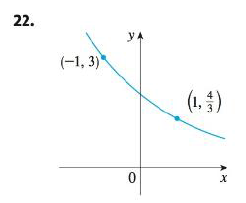Chapter 1.4, Problem 22E### Single Variable Calculus: Early Tr...

8th Edition
James Stewart
ISBN: 9781305270343

#### Solutions

Chapter
Section### Single Variable Calculus: Early Tr...

8th Edition
James Stewart
ISBN: 9781305270343
Textbook Problem

# Find the exponential function f(x) = Cbx whose graph is given.To determine

To find: The exponential function which is of the form f(x)=Cbx for the given graph.

Explanation

It is given that the equation of the given graph is of the form f(x)=Cbx .

Here, the graph represents a curve which passes through the points (−1, 3) and (1,43) .

Substitute these points in f(x)=Cbx ,

3=Cb1

3b=C (1).

43=Cb (2).

Substitute equation (1) in equation (2) and obtain the value of b as follows.

43=(3b)b4=9b2b2=49b=±23

Ignore the negative value of b as it cannot take the negative values

### Still sussing out bartleby?

Check out a sample textbook solution.

See a sample solution

#### The Solution to Your Study Problems

Bartleby provides explanations to thousands of textbook problems written by our experts, many with advanced degrees!

Get Started

#### In Exercises 9 and 10, find the limit. limx3(2x23x+4)

Calculus: An Applied Approach (MindTap Course List)

#### In Problems 1 and 2, factor out the common monomial factor. 2.

Mathematical Applications for the Management, Life, and Social Sciences